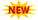WBSSC Krishi Prayukti Sahayak Recruitment || TSSPDCL AE Notification || PPSC Naib Tehsildar Admit Card ||

# NCERT Solutions For Class 5 Maths – Chapter Wise Solutions

NCERT Solutions For Class 5 Maths has been developed for students who are searching for reliable solutions for Maths problems. Our subject experts team constructed this NCERT Solutions comprehensively and carefully. We have given detailed step-by-step explanations for all problems, wherever necessary. These NCERT Solutions For Class 5 Maths has been created according to the latest CBSE (NCERT) revised syllabus. Students can grasp concepts easily and understand quickly.

## NCERT Solutions Class 5 Maths – CBSE Class 5 Maths Solutions

NCERT Solutions Class 5 Maths are given here for all chapters in the following section. Students can access these solutions by clicking on the chapter wise links given below. Class 5 NCERT Maths Book explains all of the basic concepts like large numbers, factors, tests of divisibility, fractions, averages etc.

## NCERT Solutions For Class 5 Maths – All Chapters

• Chapter 1 The Fish Tale
• Chapter 2 Shapes And Angles
• Chapter 3 How Many Squares?
• Chapter 4 Parts And Wholes
• Chapter 5 Does it Look The Same?
• Chapter 6 Be My Multiple, I’ll Be Your Factor
• Chapter 7 Can You See The Pattern?
• Chapter 8 Mapping Your Way
• Chapter 9 Boxes And Sketches
• Chapter 10 Tenths And Hundredths
• Chapter 11 Area and Its Boundary
• Chapter 12 Smart Charts
• Chapter 13 Ways To Multiply And Divide
• Chapter 14 How Big? How Heavy?

### NCERT Solutions For Class 5th Maths

We have given the easiest method to solve the questions. All of the Class 5 NCERT Solutions Maths are explained with diagrams wherever necessary. Formulas used in solving the NCERT Class 5 Maths Solutions are noted in the side wise for every problem. Therefore, practicing questions and answers in our study material will not only help students improve their Maths knowledge as well.